• ## 排序算法系列：基数排序

万次阅读 多人点赞 2016-06-16 23:14:07
这也是基数排序的魅力所在，基数排序可以理解成是建立在“计数排序”的基础之上的一种排序算法。在实际项目中，如果对效率有所要求，而不太关心空间的使用时，我会选择用计数排序（当然还有一些其他的条件），或是...
引言
今天要说的这个排序算法很特殊，它不需要直接对元素进行相互比较，也不需要将元素相互交换，你需要做的就是对元素进行“分类”。这也是基数排序的魅力所在，基数排序可以理解成是建立在“计数排序”的基础之上的一种排序算法。在实际项目中，如果对效率有所要求，而不太关心空间的使用时，我会选择用计数排序（当然还有一些其他的条件），或是一些计数排序的变形。
版权说明
著作权归作者所有。
商业转载请联系作者获得授权，非商业转载请注明出处。
本文作者：Q-WHai
发表日期： 2016年6月16日
本文链接：https://qwhai.blog.csdn.net/article/details/51695211
来源：CSDN
更多内容：分类 >> 算法与数学
基数排序
数据背景
在基数排序中，我们不能再只用一位数的序列来列举示例了。一位数的序列对基数排序来说就是一个计数排序。
这里我们列举无序序列 T = [ 2314, 5428, 373, 2222, 17 ]
排序原理
上面说到基数排序不需要进行元素的比较与交换。如果你有一些算法的功底，或者丰富的项目经验，我想你可能已经想到了这可能类似于一些“打表”或是哈希的做法。而计数排序则是打表或是哈希思想最简单的实现。
计数排序
计数排序的核心思想是，构建一个足够大的数组 hashArray[]，数组大小需要保证能够把所有元素都包含在这个数组上 。
假设我们有无序序列 T = [ 2314, 5428, 373, 2222, 17 ]
首先初始化数组 hashArray[] 为一个全零数组。当然，在 Java 里，这一步就不需要了，因为默认就是零了。
在对序列 T 进行排序时，只要依次读取序列 T 中的元素，并修改数组 hashArray[] 中把元素值对应位置上的值即可。这一句有一些绕口。打个比方，我们要把 T 映射到 hashArray[] 中，就是 hashArray[T] = 1. 也就是 hashArray = 1. 如果序列 T 中有两个相同元素，那么在  hashArray 的相应位置上的值就是 2。
下图是计数排序的原理图：
（假设有无序序列：[ 5, 8, 9, 1,  4, 2, 9, 3, 7, 1, 8, 6, 2, 3, 4, 0, 8 ]）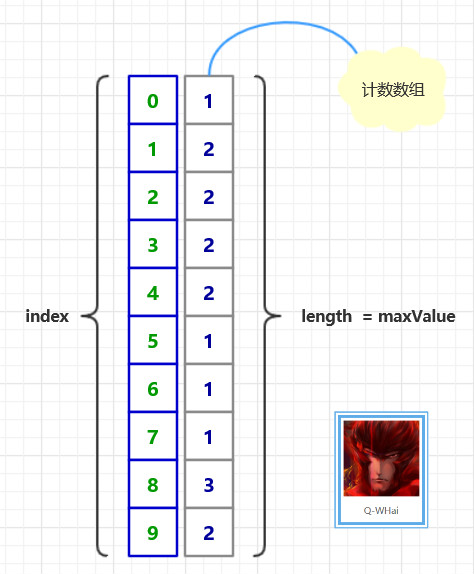基数排序原理图
上面的计数排序只是一个引导，好让你可以循序渐进地了解基数排序。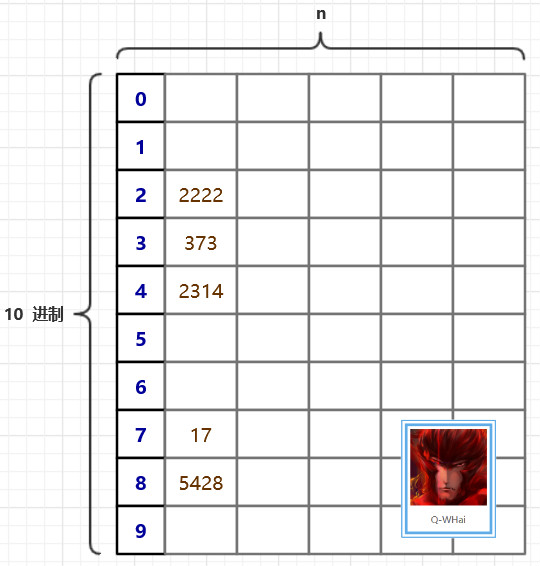上面这幅图，或许你已经在其他的博客里见到过。这是一个很好的引导跟说明。在基数排序里，我们需要一个很大的二维数组，二维数组的大小是 （10 * n）。10 代表的是我们每个元素的每一位都有 10 种可能，也就是 10 进制数。在上图中，我们是以每个数的个位来代表这个数，于是，5428 就被填充到了第 8 个桶中了。下次再进行填充的时候，就是以十位进行填充，比如 5428 在此时，就会选择以 2 来代表它。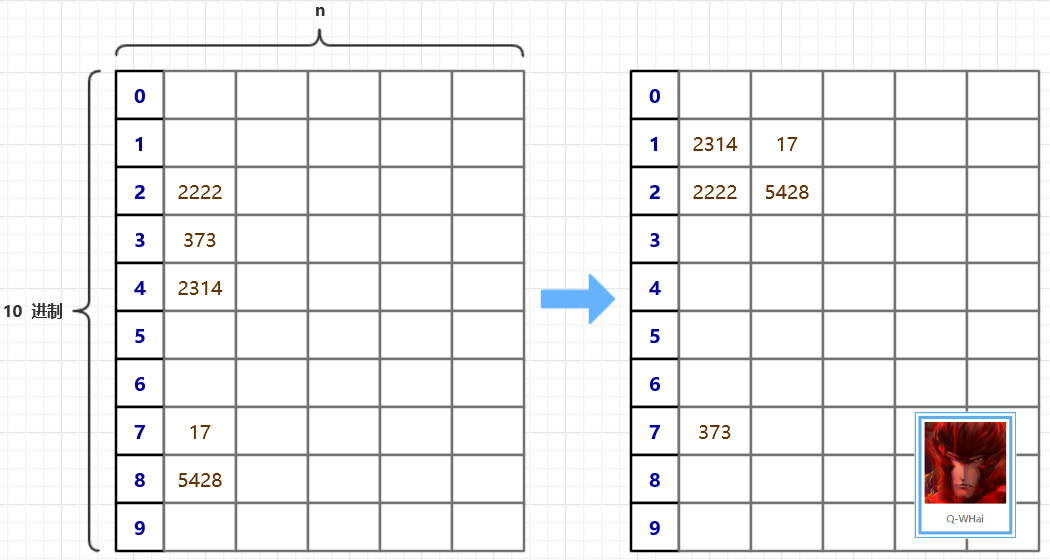算法优化
在算法的原理中，我们是以一张二维数组的表来存储这些无序的元素。使用二维数组有一个很明显的不足就是二维数组太过稀疏。数组的利用率为 10%。
在寻求优化的路上，我们想到一种可以压缩空间的方法，且时间复杂度并没有偏离得太厉害。那就是设计了两个辅助数组，一个是 count[]，一个是 bucket[]。count 用于记录在某个桶中的最后一个元素的下标，然后再把原数组中的元素计算一下它应该属于哪个“桶”，并修改相应位置的 count 值。直到最大数的最高位也被添加到桶中，或者说，当所有的元素都被被在第 0 个桶中，基数排序就结束了。
优化后的原理图如下：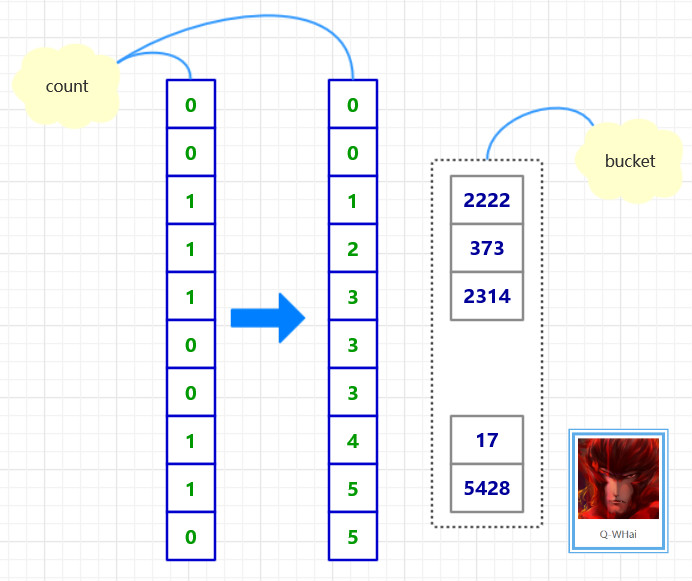算法实现
import org.algorithm.array.sort.interf.Sortable;

/**
* <p>
* 基数排序/桶排序
* </p>
* 2016年1月19日
*
* @author <a href="http://weibo.com/u/5131020927">Q-WHai</a>
* @see <a href="http://blog.csdn.net/lemon_tree12138">http://blog.csdn.net/lemon_tree12138</a>
* @version 0.1.1
*/
public class RadixSort implements Sortable {

@Override
public int[] sort(int[] array) {
if (array == null) {
return null;
}

int maxLength = maxLength(array);

return sortCore(array, 0, maxLength);
}

private int[] sortCore(int[] array, int digit, int maxLength) {
if (digit >= maxLength) {
return array;
}

final int radix = 10; // 基数
int arrayLength = array.length;
int[] bucket = new int[arrayLength];

// 统计将数组中的数字分配到桶中后，各个桶中的数字个数
for (int i = 0; i < arrayLength; i++) {
count[getDigit(array[i], digit)]++;
}

// 将各个桶中的数字个数，转化成各个桶中最后一个数字的下标索引
for (int i = 1; i < radix; i++) {
count[i] = count[i] + count[i - 1];
}

// 将原数组中的数字分配给辅助数组 bucket
for (int i = arrayLength - 1; i >= 0; i--) {
int number = array[i];
int d = getDigit(number, digit);
bucket[count[d] - 1] = number;
count[d]--;
}

return sortCore(bucket, digit + 1, maxLength);
}

/*
* 一个数组中最大数字的位数
*
* @param array
* @return
*/
private int maxLength(int[] array) {
int maxLength = 0;
int arrayLength = array.length;
for (int i = 0; i < arrayLength; i++) {
int currentLength = length(array[i]);
if (maxLength < currentLength) {
maxLength = currentLength;
}
}

return maxLength;
}

/*
* 计算一个数字共有多少位
*
* @param number
* @return
*/
private int length(int number) {
return String.valueOf(number).length();
}

/*
* 获取 x 这个数的 d 位数上的数字
* 比如获取 123 的 0 位数,结果返回 3
*
* @param x
* @param d
* @return
*/
private int getDigit(int x, int d) {
int a[] = { 1, 10, 100, 1000, 10000, 100000, 1000000, 10000000, 100000000, 1000000000 };
return ((x / a[d]) % 10);
}
}

基数排序过程图
如果我们的无序是 T = [ 2314, 5428, 373, 2222, 17 ]，那么其排序的过程就如下两幅所示。
基数排序过程图-1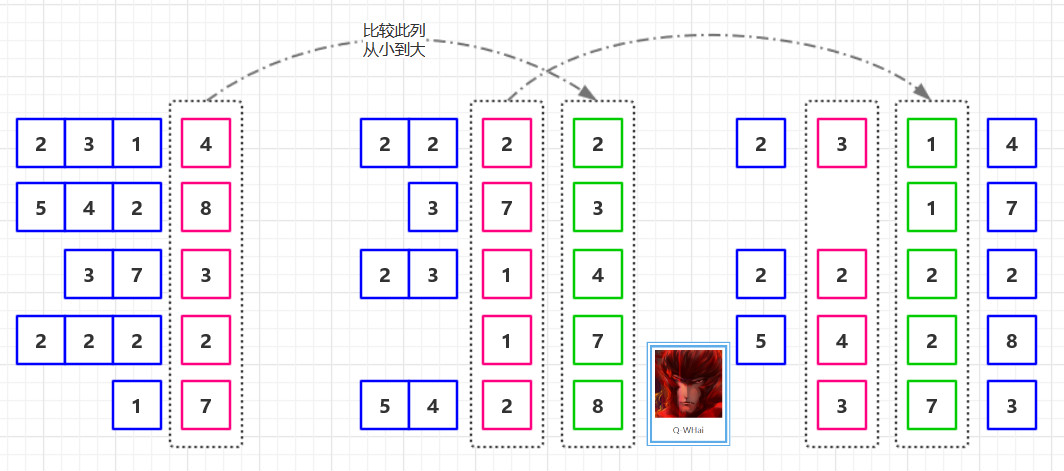基数排序过程图-2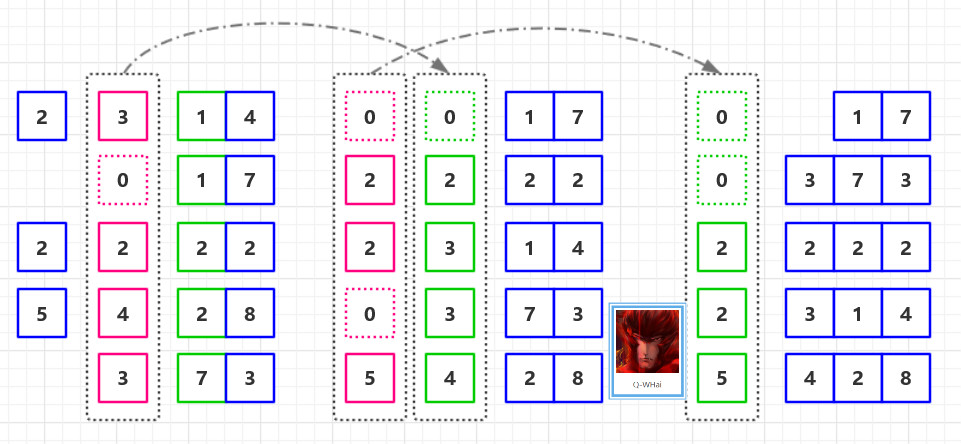复杂度分析
排序方法
时间复杂度
空间复杂度
稳定性
复杂性
平均情况
最坏情况
最好情况
基数排序
O(d*(n+r))
O(d*(n+r))
O(d*(n+r))
O(n+r)
稳定
较复杂

其中，d 为位数，r 为基数，n 为原数组个数。
在基数排序中，因为没有比较操作，所以在复杂上，最好的情况与最坏的情况在时间上是一致的，均为 O(d * (n + r))。
Ref
《算法导论》
https://github.com/qwhai/algorithms-sort
征集
如果你也需要使用ProcessOn这款在线绘图工具，可以使用如下邀请链接进行注册：https://www.processon.com/i/56205c2ee4b0f6ed10838a6d


展开全文• ## 排序

2018-06-26 16:54:56
1 插入排序 1.1 直接插入排序 1.2 希尔排序 2 选择排序 2.1 选择排序 2.2 堆排序 3 交换排序 3.1 冒泡排序 3.2 快速排序 4 归并排序 5 计数排序和基数排序...
1 插入排序
1.1 直接插入排序
1.1.1 原理分析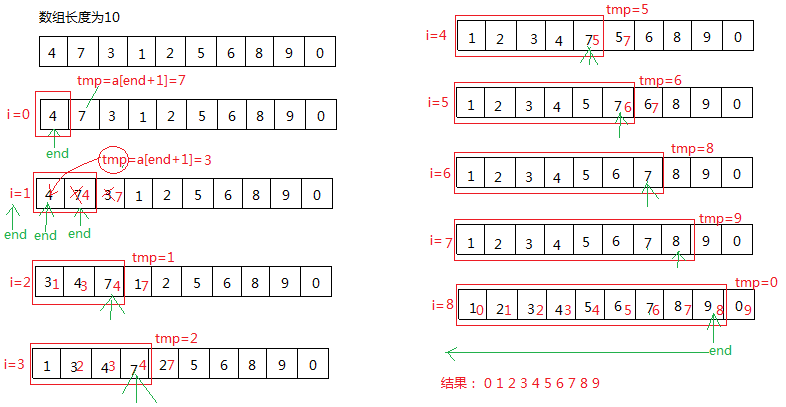1.1.2  代码实现

//直接插入排序
void InsertSort(int *a, size_t n)
{
assert(a);
for (size_t i = 0; i < n - 1; i++)
{
int end = i;
int tmp = a[end + 1];
while (end >= 0)
{
if (a[end]>tmp)
{
a[end + 1] = a[end];
end--;
}
else
break;
}
a[end + 1] = tmp;
}
}

测试结果：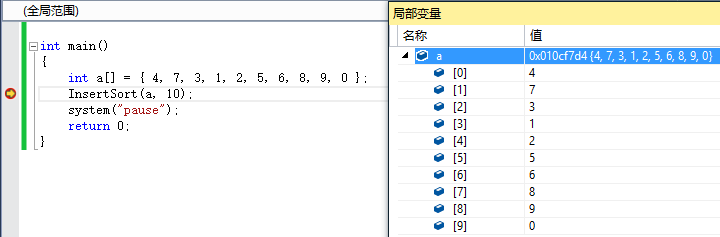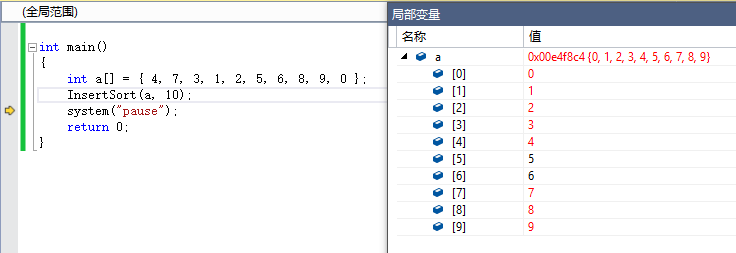1.2 希尔排序
1.2.1 原理分析
希尔排序是基于插入排序的以下两点性质而提出改进方法的：
1）插入排序在对几乎已经排好序的数据操作时，效率高，即可以达到线性排序的效率。
2）但插入排序一般来说是低效的，因为插入排序每次只能将数据移动一位。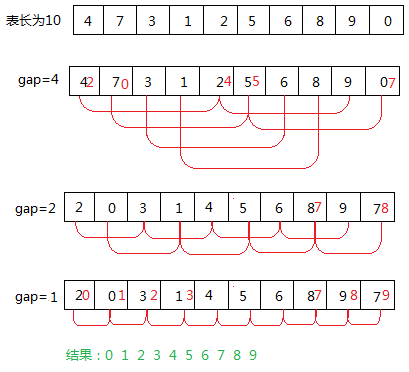1.2.2 代码实现

//1.gap>1，预排序
//2.gap=1，直接插入排序
void ShellSort(int *a,size_t n )
{
assert(a);
//预排序
int gap = n;//增量
while (gap >= 1)
{
gap = gap / 3 + 1;
for (size_t i = 0; i < n - gap; ++i)
{
int end = i;
int tmp = a[end + gap];
while (end >= 0 && a[end]>tmp)
{
a[end + gap] = a[end];
end = end - gap;
}
a[end + gap] = tmp;
}
gap--;
}
}

测试结果：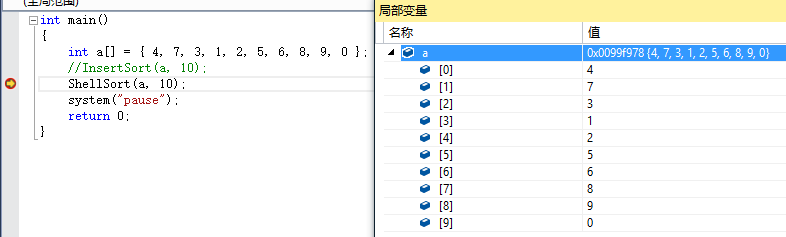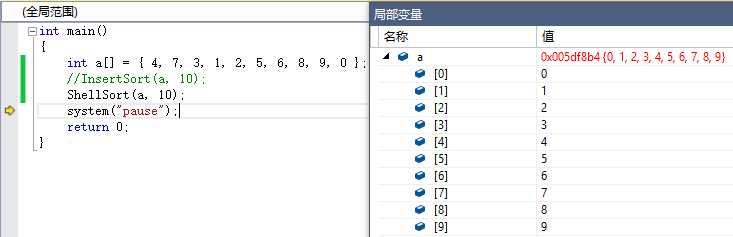2 选择排序
2.1 选择排序
2.1.1 原理分析
从n个元素中选出最小的放在最左边，选出最大的放在最右边，再对除过最左最右的元素进行上述操作。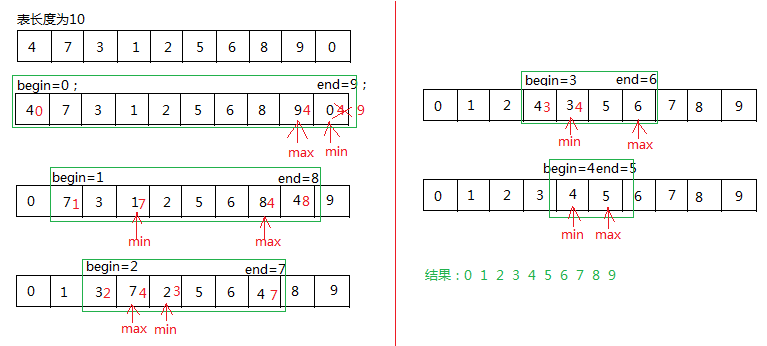2.1.2 代码实现

void SelectSort(int *a, size_t n)
{
assert(a);
int begin = 0;
int end = n - 1;
while (begin < end)
{
int min = begin;
int max = end;
for (int i = begin; i <= end; i++)
{
if (a[i] < a[min])
{
min = i;
}
else if (a[i]>a[max])
{
max = i;
}
}
swap(a[begin], a[min]);
if (max == begin)
{
max = min;
}
swap(a[max], a[end]);
begin++;
end--;
}
}

运行结果：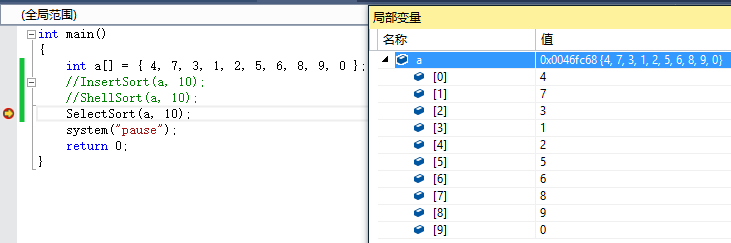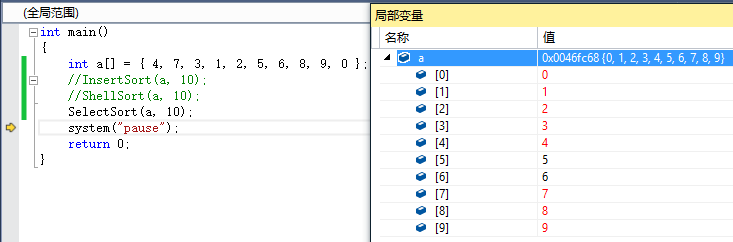2.2 堆排序
2.2.1 原理分析
1）将初始待排序关键字序列(R1,R2….Rn)构建成大堆，此堆为初始的无序区
2）将堆顶元素R与最后一个元素R[n]交换，此时得到新的无序区(R1,R2,……Rn-1)和新的有序区(Rn)
3）由于交换后新的堆顶R可能违反堆的性质，因此需要对当前无序区(R1,R2,……Rn-1)调整为新堆，然后再次将R与无序区最后一个元素交换，得到新的无序区(R1,R2….Rn-2)和新的有序区(Rn-1,Rn)。不断重复此过程直到有序区的元素个数为n-1，则整个排序过程完成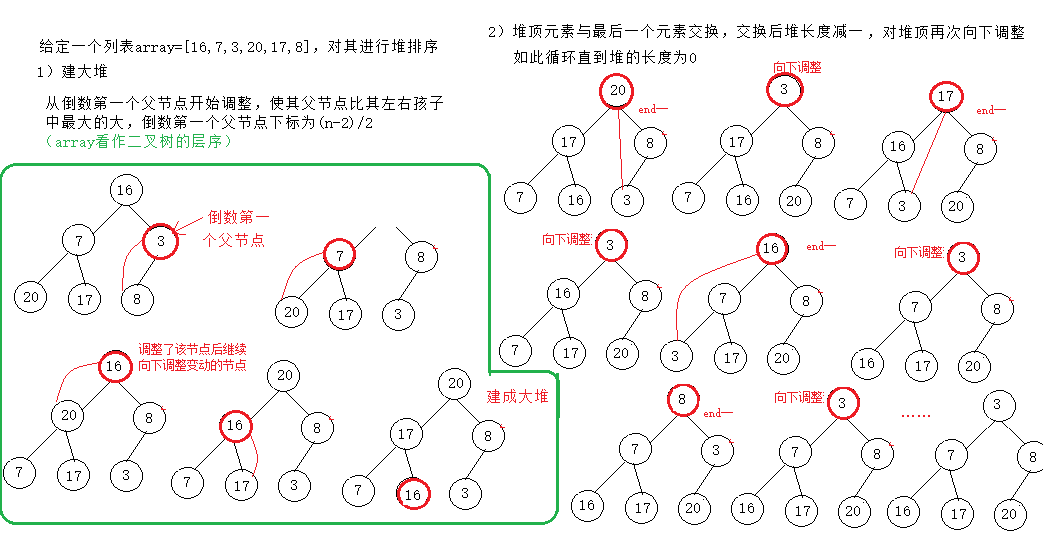2.2.2 代码实现

void AdjustDown(int* heap, int k, int root)
{
assert(heap);
for (int i = root; i >= 0; i--)
{
int parent = i;
int child = i * 2 + 1;
while (child < k)
{
if (child+1<k&&heap[child]<heap[child + 1])
{
child++;
}
if (heap[parent]<heap[child])
{
swap(heap[parent], heap[child]);
parent = child;
child = parent * 2 + 1;
}
else
{
break;
}
}

}
}
void HeapSort(int* a, int n)
{
assert(a);
for (int i = (n - 2) / 2; i >= 0; i--)
{
}
int end = n - 1;
while (end > 0)
{
swap(a,a[end]);
end--;
}
}


3 交换排序
3.1 冒泡排序
3.1.1 原理分析
冒泡排序：依次比较相邻的数据，将最大的数据放在最后；即第一趟先比较第1个和第2个数，大数在后，小数在前，再比较第2个数与第3个数，大数在后，小数在前，以此类推则将最大的数”滚动”到最后一个位置；第二趟则将次大的数滚动到倒数第二个位置……第n-1(n为无序数据的个数)趟即能完成排序。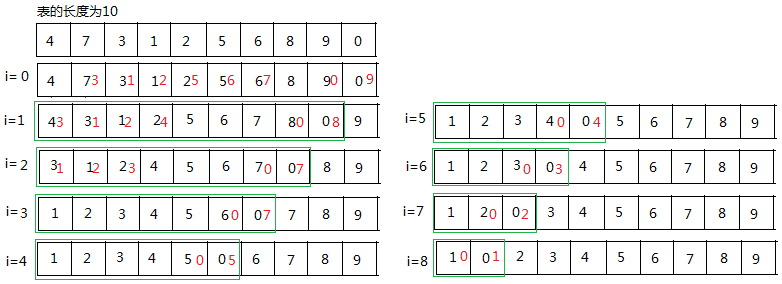优化方法：对冒泡排序算法进行简单的优化，用一个标记来记录在一趟的比较过程中是否存在交换，如果不存在交换则整个数组已经有序退出排序过程，反之则继续进行下一趟的比较。
3.1.2 代码实现

void BubbleSort(int* a, int n)
{
assert(a);
for (int i = 0; i < n - 1; ++i)
{
for(int j = 0; j < n - 1 - i; ++j)
{
if (a[j]>a[j + 1])
{
swap(a[j], a[j + 1]);
}
}
}
}

运行结果：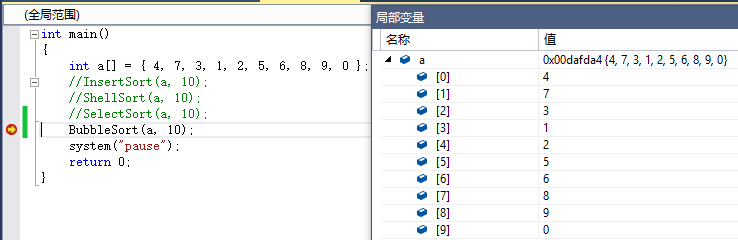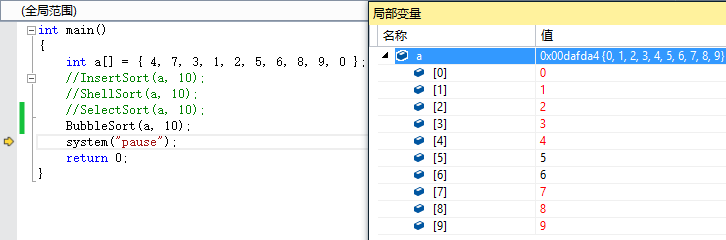优化后的：

void BubbleSort(int* a, int n)
{
assert(a);
bool flag = true;
for (int i = 0; i < n - 1; ++i)
{
for(int j = 0; j < n - 1 - i; ++j)
{
if (a[j]>a[j + 1])
{
flag = false;
swap(a[j], a[j + 1]);
}
}
if (flag == true)
break;
}
}

3.2 快速排序
快速排序使用分治的思想，通过一趟排序将待排序列分割成两部分，其中一部分记录的关键字均比另一部分记录的关键字小。之后分别对这两部分记录继续进行排序，以达到整个序列有序的目的。
步骤：

1）先从数列中取出一个数作为基准数
2）分区过程，将比这个数大的数全放到它的右边，小于或等于它的数全放到它的左边
3）再对左右区间重复第二步，直到各区间只有一个数

快速排序的三种方法：1）左右指针法；2）挖坑法；3）前后指针法
递归方法：
3.2.1 左右指针法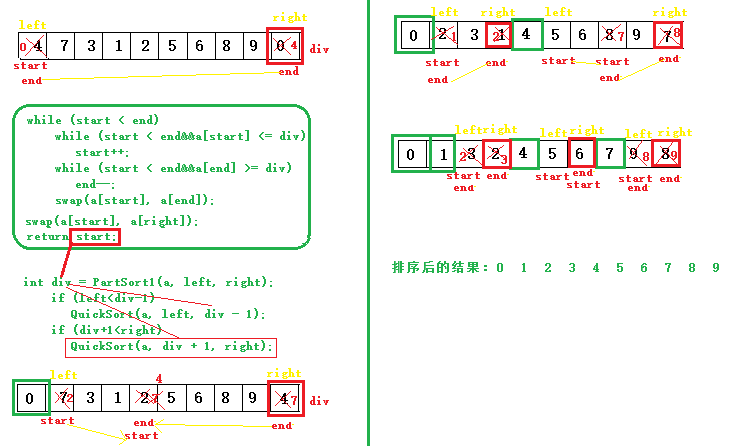int PartSort1(int* a, int left, int right)
{
assert(a != NULL);
int start = left;
int end = right;
int div = a[end];
while (start < end)
{
while (start < end&&a[start] <= div)
{
start++;
}
while (start < end&&a[end] >= div)
{
end--;
}
swap(a[start], a[end]);
}
swap(a[start], a[right]);
return start;
}
void QuickSort(int* a, int left,int right)
{
if (left >= right)
{
return;
}
int div = PartSort1(a, left, right);
if (left<div-1)
QuickSort(a, left, div - 1);
if (div+1<right)
QuickSort(a, div + 1, right);
}

3.2.2 挖坑法

int PartSort2(int* a, int left, int right)
{
assert(a != NULL);
int start = left;
int end = right;
int div = a[end];
while (start < end)
{
while (start < end&&a[start] <= div)
{
start++;
}
if (start < end)
a[end--] = a[start];
while (start < end&&a[end] >= div)
{
end--;
}
if (start < end)
a[start++] = a[end];
}
a[start] = div;
return start;
}
void QuickSort(int* a, int left, int right)
{
if (left >= right)
{
return;
}
int div = PartSort2(a, left, right);
if (left < div - 1)
QuickSort(a, left, div - 1);
if (div + 1 < right)
QuickSort(a, div + 1, right);
}

3.2.3 前后指针法

int PartSort3(int* a, int left, int right)
{
assert(a != NULL);
int div = a[right];
int cur = left;
int prev = left-1;
while (cur < right)
{
if (a[cur] <= div&&++prev != cur)
swap(a[cur], a[prev]);
cur++;
}
if (a[++prev]!=div)
swap(a[prev], a[right]);
return prev;
}
void QuickSort(int* a, int left, int right)
{
if (left >= right)
{
return;
}
int div = PartSort3(a, left, right);
if (left < div - 1)
QuickSort(a, left, div - 1);
if (div + 1 < right)
QuickSort(a, div + 1, right);
}

3.2.4 优化方法：三数取中法
快速排序是一种交换类的排序，它同样是分治法的经典体现。在一趟排序中将待排序的序列分割成两组，其中一部分记录的关键字均小于另一部分。然后分别对这两组继续进行排序，以使整个序列有序。在分割的过程中，枢纽值的选择至关重要，本文采取了三位取中法，可以很大程度上避免分组”一边倒”的情况。快速排序平均时间复杂度也为O(nlogn)

int GetMidIndex(int* a, int left, int right)
{
assert(a);
if (left >= right)
return -1;
int mid = left + ((right - left) >> 1);
if (a[right] > a[left])
{
if (a[right] < a[mid])
{
return right;
}
else if (a[left] > a[mid])
{
return left;
}
else
{
return mid;
}
}
else
{
if (a[mid] > a[left])
{
return left;
}
else if (a[right] > a[mid])
{
return right;
}
else
{
return mid;
}
}
}
int PartSort(int* a, int left, int right)
{
assert(a != NULL);
int div = GetMidIndex(a,left,right);
int key = a[div];
swap(a[div], a[right]);
int cur = left;
int prev = left-1;
while (cur < right)
{
if (a[cur] <= key&&++prev != cur)
{
swap(a[cur], a[prev]);
}
cur++;
}
swap(a[++prev], a[right]);
return prev;
}
void QuickSort(int* a, int left, int right)
{
if (left >= right)
{
return;
}
//当区域很小的时候，插入排序比快排递归效率高
if (right - left <= 20)
{
InsertSort(a + left, right - left + 1);
}
int div = PartSort(a, left, right);
if (left < div - 1)
QuickSort(a, left, div - 1);
if (div + 1 < right)
QuickSort(a, div + 1, right);
}


3.2.5  非递归法

int PartSort1(int* a, int left, int right)
{
assert(a != NULL);
int start = left;
int end = right;
int div = a[end];
while (start < end)
{
while (start < end&&a[start] <= div)
{
start++;
}
while (start < end&&a[end] >= div)
{
end--;
}
swap(a[start], a[end]);
}
swap(a[start], a[right]);
return start;
}
void QuickSortNOR(int* a, int left, int right)
{
stack<int> S;
if (left < right)
{
S.push(right);
S.push(left);
}
while (!S.empty())
{
int start = S.top();
S.pop();
int end = S.top();
S.pop();
int div = PartSort1(a, start, end);
if (start < div - 1)
{
S.push(div-1);
S.push(start);
}
if (div + 1 < end)
{
S.push(end);
S.push(div + 1);
}
}
}

4 归并排序
4.1 原理
归并排序（MERGE-SORT）是利用归并的思想实现的排序方法，该算法采用经典的分治（divide-and-conquer）策略（分治法将问题分(divide)成一些小的问题然后递归求解，而治(conquer)的阶段则将分的阶段得到的各答案”修补”在一起，即分而治之)。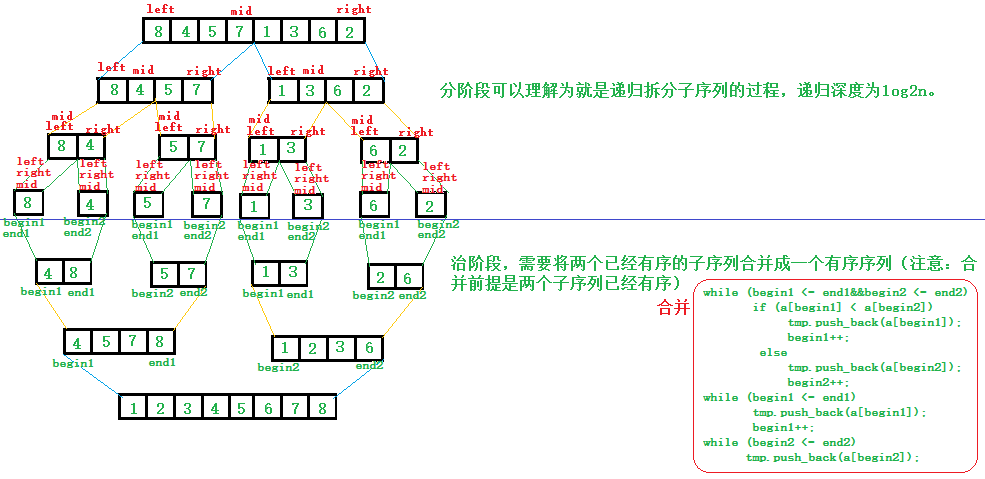4.2 代码实现

void _MergeSort(int* a,int left,int right,vector<int> tmp)
{
if (left >= right)
{
return;
}
assert(a);
int mid = left + ((right - left) >> 1);
//划分子区间
_MergeSort(a, left, mid,tmp);
_MergeSort(a, mid+1,right,tmp);
//归并
int begin1 = left;
int end1 = mid;
int begin2 = mid + 1;
int end2 = right;
while (begin1 <= end1&&begin2 <= end2)
{
if (a[begin1] < a[begin2])
{
tmp.push_back(a[begin1]);
begin1++;
}
else
{
tmp.push_back(a[begin2]);
begin2++;
}
}
while (begin1 <= end1)
{
tmp.push_back(a[begin1]);
begin1++;
}
while (begin2 <= end2)
{
tmp.push_back(a[begin2]);
begin2++;
}
int index = 0;
while (left <= right){
a[left++] = tmp[index++];
}
}


5 非比较排序（计数排序和基数排序）
5.1 计数排序
5.1.1 原理
给定一组要排序的序列，找出这组序列中的最大值，然后开辟一个最大值加1大小的数组，将这个数组里面的元素全部置零，然后用这个数组统计出要排序的序列中各个元素出现的次数。等到统计完成的时候，排序就已经完成了。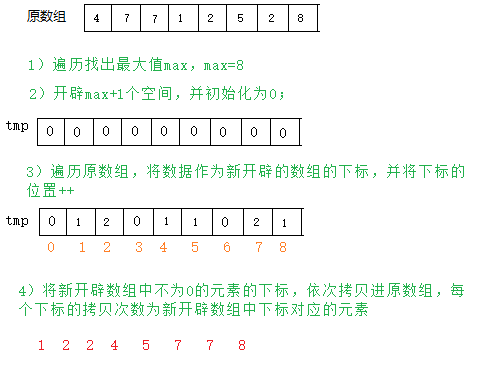算法优化：
计数排序适用于数据比较集中的序列排序。
如果是序列56 ，77，82，则开辟的数组下标56以前的空间就浪费了，基于节省空间的角度进行考虑，可以开辟更小的临时数组统计次数。
方法：找出要排序的这组元素中的最大值和最小值，这样就确定了这组元素的范围，然后开辟这个范围加1大小的数组，然后再将要排序的元素映射到这个新开辟的数组中就可以了。

5.1.2 代码实现
简单实现：

int GetMaxNum(int* a, int n)
{
assert(a != NULL);
int ret = a;
for (int i = 1; i < n; i++)
{
if (a[i]>ret)
{
ret = a[i];
}
}
return ret;
}
void Count_Sort(int* a, int n)
{
assert(a != NULL);
int Max = GetMaxNum(a, n);
int * tmp = new int[Max + 1];
memset(tmp, 0, sizeof(int)*(Max + 1));
for (int i = 0; i < n; i++)
{
tmp[a[i]]++;
}
int index = 0;
for (int i = 0; i < Max + 1; i++)
{
while (tmp[i] != 0)
{
a[index++] = i;
tmp[i]--;
}
}
delete tmp;
}

优化代码实现：

int GetLength(int* a, int n)
{
assert(a != NULL);
int ret = 0;
int max = 0;
int min = 0;
for (int i = 1; i < n; i++)
{
if (a[i]>a[max])
{
max = i;
}
if (a[i] < a[min])
{
min = i;
}
}
ret = a[max] - a[min]+1;
return ret;
}
void Count_Sort(int* a, int n)
{
assert(a != NULL);
int len = GetLength(a, n);
int * tmp = new int[len];
memset(tmp, 0, sizeof(int)*len);
for (int i = 0; i < n; i++)
{
tmp[a[i]]++;
}
int index = 0;
for (int i = 0; i < len; i++)
{
while (tmp[i] != 0)
{
a[index++] = i;
tmp[i]--;
}
}
delete tmp;
}

5.2 基数排序
5.2.1 原理
构建一个足够大的数组Array[]，数组大小需要保证能够把要排序的所有元素都包含在这个数组上 。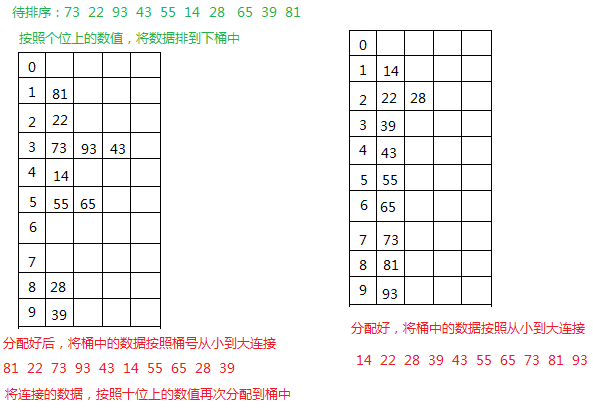从上述可以看到，分别根据数据的个位，十位等进行排序，可知数据排序次数为数据的最大长度。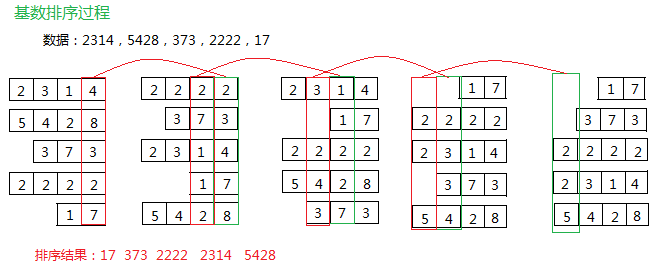优化方法：
以一张二维数组的表来存储这些无序的元素，使用二维数组有一个很明显的不足就是二维数组太过稀疏。数组的利用率为 低。
可用一种压缩空间的方法，且时间复杂度并没有偏离得太厉害。设计两个辅助数组，一个是 count[]，一个是 bucket[]。count 用于记录数据在 bucket[]中的的下标，然后再计算属于bucket[]的位置，并修改相应位置的 count 值。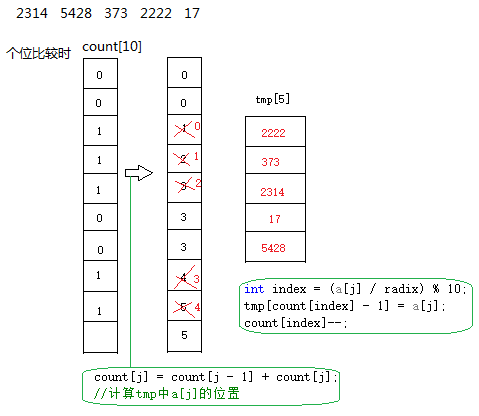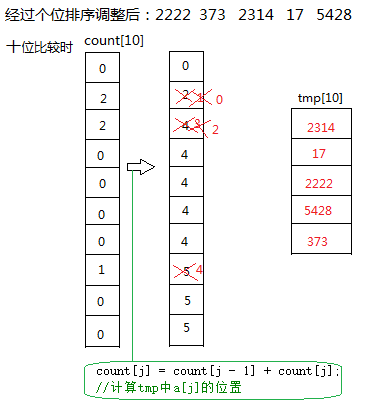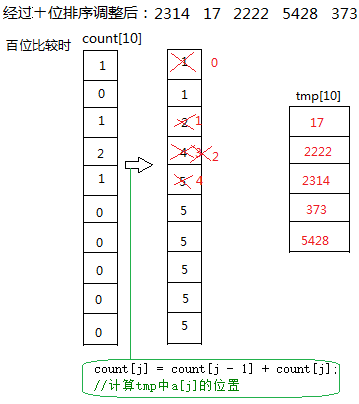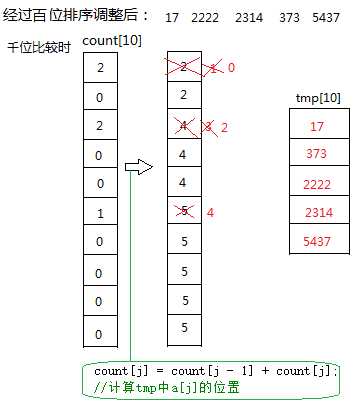5.2.2 代码实现

#define N 10
int GetMaxbit(int* a, int n)//求数组中最大位数
{
int bit = 1;
int p = 10;
for (int i = 0; i < n; i++)
{
if (a[i] >= p)
{
bit++;
p = p * 10;
}
}
return bit;
}
void CountSort(int* a, int n)
{
if (a == NULL||n<1)
{
return;
}
int bit = GetMaxbit(a, n);
int count;
int tmp[N];
for (int i = 1; i <= bit; ++i)
{
for (int j = 0; j < 10; j++)
count[j] = 0; //每次分配前清空计数器
for (int j = 0; j < n; j++)
{
int index = (a[j] / radix) % 10;
count[index]++;
}
for (int j = 1; j < 10; j++)
count[j] = count[j - 1] + count[j]; //将tmp中a[j]的位置

for (int j = n - 1; j >= 0; j--) //将所有桶中记录依次收集到tmp中
{
int index = (a[j] / radix) % 10;
tmp[count[index] - 1] = a[j];
count[index]--;
}
for (int j = 0; j < n; j++) //将临时数组的内容复制到data中
a[j] = tmp[j];
}
}


总：排序算法性能分析

类别
排序方法
时间复杂度(平均情况)
时间复杂度(最好情况)
时间复杂度(最坏情况)
空间复杂度
稳定性

插入排序
插入排序
O(N^2)
O(N)
O(N^2)
O(1）
稳定

插入排序
shell排序
O(N^1.3)
O(N)
O(N^2)
O(1）
不稳定

选择排序
选择排序
O(N^2)
O(N^2)
O(N^2)
O(1）
不稳定

选择排序
堆排序
O(N* logN)
O(N *logN)
O(N *logN)
O(1）
不稳定

交换排序
冒泡排序
O(N^2)
O(N)
O(N^2)
O(1）
稳定

交换排序
快速排序
O(N* logN)
O(N *logN)
O(N *2)
O(logN)
不稳定

归并排序
归并排序
O(N* logN)
O(N *logN)
O(N *logN)
O(N)
稳定

计数排序:时间复杂度:O(N), 空间复杂度O(最大数-最小数)
基数排序:时间复杂度:O(N*位数)，空间辅助度O(N)
展开全文C++ sort
• 1 算法的魅力深刻研究排序算法是入门算法较为好的一种方法，现在还记得4年前手动实现常见8种排序算法，通过随机生成一些数据，逐个校验代码实现的排序过程是否与预期的一致，越做越有劲，越有劲越...


1 算法的魅力深刻研究排序算法是入门算法较为好的一种方法，现在还记得4年前手动实现常见8种排序算法，通过随机生成一些数据，逐个校验代码实现的排序过程是否与预期的一致，越做越有劲，越有劲越想去研究，公交车上，吃饭的路上。。。那些画面，现在依然记忆犹新。能力有限，当时并没有生成排序过程的动画，所以这些年想着抽时间一定把排序的过程都制作成动画，然后分享出来，让更多的小伙伴看到，通过排序算法的动态演示动画，找到学习算法的真正乐趣，从而迈向一个新的认知领域。当时我还是用C++写的，时过境迁，Python迅速崛起，得益于Python的简洁，接口易用，最近终于有人在github中开源了使用Python动画展示排序算法的项目，真是倍感幸运。动画还是用matplotlib做出来的，这就更完美了，一边学完美的算法，一边还能提升Python熟练度，一边还能学到使用matplotlib制作动画。2 完美的答案这个库一共演示8个常见的排序算法：bubble-sort : Only show the visualization of bubble sorting algorithm in the animation. The following arguments have similar functions.comb-sortheap-sortinsertion-sortmerge-sortquick-sortselection-sortshell-sort启动的脚本是output.py，脚本的参数有三类，下面逐个解释。python output.py play heap-sort reversed
play表示展示排序的动画，其他两个选项：保存html和mp4play : Play an animation of a specific sorting algorithm or all algorithms in a new window, as a "figure" to Matplotlib.save-html : Save the animation as a HTML page with a sequence of images.save-mp4 : Save the animation as a MP4 video.heap-sort表示堆排序，就是此次执行脚本你想看哪个排序算法的动画展示,设置为quick-sort表示查看快排动画, all表示所有排序算法一次展示。reversed 这类参数是我重点想说的，这类参数还有如下其他几个选项。通常说一个快排平均时间复杂度为nlog2n，为什么是平均呢？我们很难找到一个真正100%准确的函数t，输入data，通过t(data)计算出准确的理论执行时间，因为data的分布无法准确的拟合出来，而它又直接影响到实际的排序时间，比如输入一个几乎排序好的序列，一个没有重复元素的序列，一个随机序列，一个递减序列。所以只能根据某类分布给出大概的预估执行时间值。almost-sorted : Sort an almost-sorted sequence.few-unique : Sort a few-unique sequence.random (default) : Sort a random sequence.reversed : Sort a descending sequence.3 动画展示使用的模块和实例代码如下：使用的包，主要是内置模块random, os, sys, re，以及 matplotlib的 animation功能，剩下的就是手动实现的8个排序算法。import random
import os
import sys
import re
from matplotlib import pyplot as plt
from matplotlib import animation
from sorting.data import Data
from sorting.selectionsort import selection_sort
from sorting.bubblesort import bubble_sort
from sorting.insertionsort import insertion_sort
from sorting.shellsort import shell_sort
from sorting.mergesort import merge_sort
from sorting.quicksort import quick_sort
from sorting.heapsort import heap_sort
from sorting.combsort import comb_sort
from sorting.monkeysort import monkey_sort

快速排序代码，会保存所有的操作帧：# Script Name     : quicksort.py
# Author          : Howard Zhang
# Created         : 14th June 2018
# Version         : 1.0
# Modifications	  :
# Description     : Quick sorting algorithm.

import copy
from .data import Data

def quick_sort(data_set):
# FRAME OPERATION BEGIN
frames = [data_set]
# FRAME OPERATION END
ds = copy.deepcopy(data_set)
qsort(ds, 0, Data.data_count, frames)
# FRAME OPERATION BEGIN
frames.append(ds)
return frames
# FRAME OPERATION END

if tail - head > 1:
# FRAME OPERATION BEGIN
ds_y = copy.deepcopy(ds)
ds_y[i].set_color('y')
# FRAME OPERATION END
j = tail - 1
pivot = ds[j].value
while i < j:
# FRAME OPERATION BEGIN
frames.append(copy.deepcopy(ds_y))
frames[-1][i if ds[i].value == pivot else j].set_color('r')
frames[-1][j if ds[i].value == pivot else i].set_color('k')
# FRAME OPERATION END
if ds[i].value > pivot or ds[j].value < pivot:
ds[i], ds[j] = ds[j], ds[i]
# FRAME OPERATION BEGIN
ds_y[i], ds_y[j] = ds_y[j], ds_y[i]
frames.append(copy.deepcopy(ds_y))
frames[-1][i if ds[i].value == pivot else j].set_color('r')
frames[-1][j if ds[i].value == pivot else i].set_color('k')
# FRAME OPERATION END
if ds[i].value == pivot:
j -= 1
else:
i += 1
qsort(ds, i+1, tail, frames)

我已经执行完8个排序算法，录制了3个动画，效果如下：1) 快速排序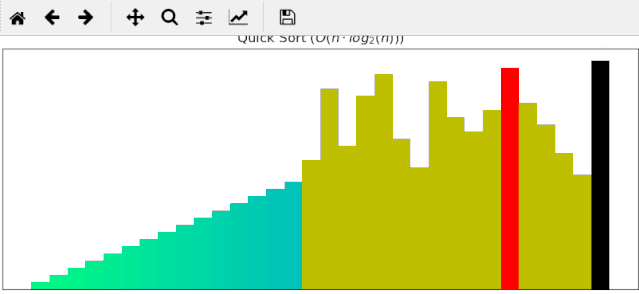2) 归并排序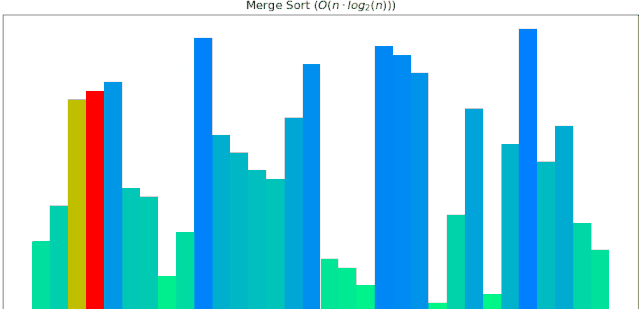3) 堆排序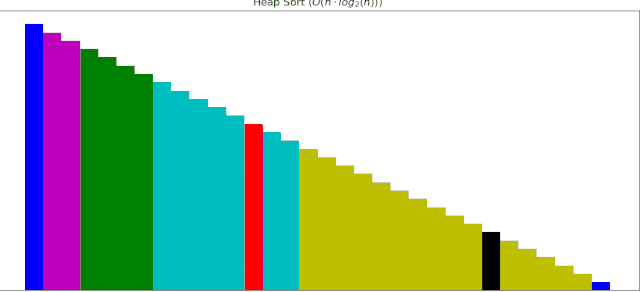项目地址，这里面有完整源码：https://github.com/zamhown/sorting-visualizer

展开全文• 本文来自作者 耀升 在 GitChat 上分享「常见的七种排序算法解析」，「阅读原文」查看交流实录 「文末高能」 编辑 | 乔巴 1. 选择排序 实现原理 首先从未排序序列中找到最小的元素，放置到排序...本文来自作者 耀升 在 GitChat 上分享「常见的七种排序算法解析」，「阅读原文」查看交流实录

「文末高能」

编辑 | 乔巴

1. 选择排序

实现原理

首先从未排序序列中找到最小的元素，放置到排序序列的起始位置，然后从剩余的未排序序列中继续寻找最小元素，放置到已排序序列的末尾。所以称之为选择排序。

代码实现案例分析时间复杂度与空间复杂度

每次要找一遍最小值，最坏情况下找 n 次，这样的过程要执行 n 次，所以时间复杂度还是 O(n^2)。空间复杂度是 O(1)。

2. 快速排序

实现原理

在数据集之中，选择一个元素作为”基准”（pivot）。

所有小于”基准”的元素，都移到”基准”的左边；所有大于”基准”的元素，都移到”基准”的右边。这个操作称为分区 （partition）。
操作，分区操作结束后，基准元素所处的位置就是最终排序后它的位置。

对”基准”左边和右边的两个子集，不断重复第一步和第二步，直到所有子集只剩下一个元素为止。

代码实现案例分析时间复杂度与空间复杂度

快速排序也是一个不稳定排序，平均时间复杂度是 O(nlogn)。空间复杂度是 O(logn)。

3. 冒泡排序

实现原理

依次比较相邻的两个元素，如果第一个元素大于第二个元素就交换它们的位置。这样比较一轮之后，最大的元素就会跑到队尾。然后对未排序的序列重复这个过程，最终转换成有序序列。

代码实现案例分析

以数组 arr = [3 4 2 8 0] 为例说明，加粗的数字表示每次循环要比较的两个数字：时间复杂度与空间复杂度

由于我们要重复执行n次冒泡，每次冒泡要执行n次比较（实际是1到n的等差数列，也就是 (a1 + an) * n / 2），也就是 O(n^2)。 空间复杂度是O(1)。

4. 插入排序

实现原理

认为第一个元素是排好序的，从第二个开始遍历。

拿出当前元素的值，从排好序的序列中从后往前找。

如果序列中的元素比当前元素大，就把它后移。直到找到一个小的。

把当前元素放在这个小的后面（后面的比当前大，它已经被后移了）。

代码实现原理图解

案例1案例2时间复杂度与空间复杂度

因为要选择 n 次，而且插入时最坏要比较n次，所以时间复杂度同样是 O(n^2)。空间复杂度是 O(1)。

5. 希尔排序

实现原理

先取一个正整数 d1(d1 < n)，把全部记录分成 d1 个组，所有距离为 d1 的倍数的记录看成一组，然后在各组内进行插入排序

然后取 d2(d2 < d1)

重复上述分组和排序操作；直到取 di = 1(i >= 1) 位置，即所有记录成为一个组，最后对这个组进行插入排序。一般选 d1 约为
n/2，d2 为 d1 /2， d3 为 d2/2 ，…， di = 1。

代码实现案例分析

假设有数组 array = [80, 93, 60, 12, 42, 30, 68, 85, 10]，首先取 d1 = 4，将数组分为 4 组，如下图中相同颜色代表一组：然后分别对 4 个小组进行插入排序，排序后的结果为：然后，取 d2 = 2，将原数组分为 2 小组，如下图：然后分别对 2 个小组进行插入排序，排序后的结果为：最后，取 d3 = 1，进行插入排序后得到最终结果：时间复杂度与空间复杂度

希尔排序的时间复杂度受步长的影响，平均时间复杂度是 O(n log2 n)，空间复杂度是 O(1)。

6. 归并排序

实现原理

把 n 个记录看成 n 个长度为 l 的有序子表

进行两两归并使记录关键字有序，得到 n/2 个长度为 2 的有序子表

重复第 2 步直到所有记录归并成一个长度为 n 的有序表为止。

总而言之，归并排序就是使用递归，先分解数组为子数组，再合并数组。

代码实现
public static int[] mergeSort(int[] arr){
int[] temp =new int[arr.length];
internalMergeSort(arr, temp, 0, arr.length-1);
return temp;
}
private static void internalMergeSort(int[] a, int[] b, int left, int right){
//当left==right的时，已经不需要再划分了
if (left<right){
int middle = (left+right)/2;
internalMergeSort(a, b, left, middle);          //左子数组
internalMergeSort(a, b, middle+1, right);       //右子数组
mergeSortedArray(a, b, left, middle, right);    //合并两个子数组
}
}
// 合并两个有序子序列 arr[left, ..., middle] 和 arr[middle+1, ..., right]。temp是辅助数组。
private static void mergeSortedArray(int arr[], int temp[], int left, int middle, int right){
int i=left;
int j=middle+1;
int k=0;
while ( i<=middle && j<=right){
if (arr[i] <=arr[j]){
temp[k++] = arr[i++];
}
else{
temp[k++] = arr[j++];
}
}
while (i <=middle){
temp[k++] = arr[i++];
}
while ( j<=right){
temp[k++] = arr[j++];
}
//把数据复制回原数组
for (i=0; i<k; ++i){
arr[left+i] = temp[i];
}
}

案例分析

案例1

以数组
array = [4 2 8 3 5 1 7 6] 为例，首先将数组分为长度为 2 的子数组，并使每个子数组有序：案例2时间复杂度与空间复杂度

在合并数组过程中，实际的操作是当前两个子数组的长度，即 2m。又因为打散数组是二分的，最终循环执行数是 logn。所以这个算法最终时间复杂度是 O(nlogn)，空间复杂度是 O(1)。

7. 堆排序

实现原理

堆排序就是把最大堆堆顶的最大数取出，将剩余的堆继续调整为最大堆，再次将堆顶的最大数取出，这个过程持续到剩余数只有一个时结束。在堆中定义以下几种操作：

最大堆调整（Max-Heapify）：将堆的末端子节点作调整，使得子节点永远小于父节点

创建最大堆（Build-Max-Heap）：将堆所有数据重新排序，使其成为最大堆

堆排序（Heap-Sort）：移除位在第一个数据的根节点，并做最大堆调整的递归运算Parent(i) = floor((i-1)/2)，i 的父节点下标

Left(i) = 2i + 1，i 的左子节点下标

Right(i) = 2(i + 1)，i 的右子节点下标

代码实现
/**
* 堆排序
*/
public static int[] heapSort(int[] arr) {
// 将待排序的序列构建成一个大顶堆
for (int i = arr.length / 2; i >= 0; i--){
}

// 逐步将每个最大值的根节点与末尾元素交换，并且再调整二叉树，使其成为大顶堆
for (int i = arr.length - 1; i > 0; i--) {
swap(arr, 0, i); // 将堆顶记录和当前未经排序子序列的最后一个记录交换
}
return arr;
}

/**
* 构建堆的过程
* @param arr 需要排序的数组
* @param i 需要构建堆的根节点的序号
* @param n 数组的长度
*/
private static void heapAdjust(int[] arr, int i, int n) {
int child;
int father;
for (father = arr[i]; leftChild(i) < n; i = child) {
child = leftChild(i);

// 如果左子树小于右子树，则需要比较右子树和父节点
if (child != n - 1 && arr[child] < arr[child + 1]) {
child++; // 序号增1，指向右子树
}

// 如果父节点小于孩子结点，则需要交换
if (father < arr[child]) {
arr[i] = arr[child];
} else {
break; // 大顶堆结构未被破坏，不需要调整
}
}
arr[i] = father;
}

// 获取到左孩子结点
private static int leftChild(int i) {
return 2 * i + 1;
}

// 交换元素位置
private static void swap(int[] arr, int index1, int index2) {
int tmp = arr[index1];
arr[index1] = arr[index2];
arr[index2] = tmp;
}

案例分析时间复杂度与空间复杂度

堆执行一次调整需要 O(logn) 的时间，在排序过程中需要遍历所有元素执行堆调整，所以最终时间复杂度是 O(nlogn)。空间复杂度是 O(1)。

近期热文

《连公式都没看懂？！学渣谨碰这个「神经网络」》

《当我说要做大数据工程师时他们都笑我，直到三个月后……》

《当年校招时，我就死在了这个问题上...》

《如何成为一个
IT 界的女装大佬？》

《技术学到多厉害，才能顺利进入BAT？》

福利「阅读原文」了解更多知识


展开全文• 我们先来总的分析分析归并和快拍这两个比较厉害排序算法 其平均时间复杂度都是nlogn 为什么是nlogn呢 其实logn是递归栈的深度，而n是遍历的个数 所以递归层数加上遍历个数总结果使得 就排序完了 时间复杂度就是...
• IOS项目中会用到对通讯录的联系人或是会员按姓名为关键字排序，因为NSArray并不直接支持对汉字的排序，这就要通过将汉字转换成拼音完成按A~Z的排序，这看起来是个头疼的问题，因为牵扯到汉字转为拼音，kmyhy给出一个...
• 我非常喜欢这个算法，看了这么多快速排序，这个快速排序由简入深，还有快速排序的改进版，真是赞叹，他们真厉害简单的从两头同步开始找轴点，我都看了好几天，好不容易理解了，发现邓俊辉老师不仅由实例，看不...
• //快排为非稳定排序，因为...//快排例程很多，自我感觉全面可靠的是黑皮书教材上的，老美就是厉害，这一点确实得佩服人家，国内的计算机教材真心不敢恭维 //几个快排例程： //一：教材（赞一个） int Median3(int arr
• 冒泡排序是交换排序，它是非常慢的，比起几种简单排序，例如选择，插入排序。冒泡排序的时间性能要差的多。因为总要交换数据，只是两个两个的比，不仅逻辑判断多，交换数据的频次也高。  但交换排序就如此失败吗。...
• 上篇博客“排序算法(三) - 交换排序”介绍了交换排序，这篇博客咱们聊聊选择排序； 选择排序（Selection Sorting）的基本...如果不是，你就比我厉害，因为刚开始的时候真心看不懂，后来动手排序一下也就明白了，其实很
• js系列教程5-数据结构和算法全解js排序算法详解-插入排序插入排序的原理其实很好理解，可以类比选择排序。选择排序时在两个空间进行，等于说每次从旧的空间选出最值放到新的空间，而插入排序则是在同一空间进行。...数据结构 算法 js
• 生活中这种事很常见，比如斗地主，如果是一张牌一张牌地拿在手上的话，普通人的大小王永远是放在两端（这取决于每个人的排序习惯）。 如上图所示，这时候你拿起了一张7，左手上的牌是排好序的，现在JavaScript 算法
• ## 简单排序--快速排序

千次阅读 热门讨论 2016-05-22 16:56:30
不知亲爱的小伙伴们是否发现，快速排序的交换是跳跃式的，交换的距离变大了很多了，不像冒泡排序那样是相邻的排序，这样效率就提高了很多。
• 每次看算法书的第一章往往都是排序……所以每次看排序我都有点害怕，因为算法真的超级难 不过还是躲不过的坎的啦，既然现在写的题开始要求时间复杂度了，那么算法肯定也不能省的了……总不能真的跟搬砖一样对吧 ...算法
• 1.桶排序(Bucket Sort) 基本思路是： 将待排序元素划分到不同的痛。先扫描一遍序列求出最大值 maxV 和最小值 minV ， 设桶的个数为 k ，则把区间 [minV, maxV] 均匀划分成 k 个区间，每个区间就是一个桶。将序列中的...
• 希尔排序（ShellSort）是以它的发明者Donald Shell名字命名的，希尔排序是插入排序的改进版，实现简单，对于中等规模数据的性能表现还不错排序思想前情回顾：强...
• 插入排序简单的一种排序方法，对于少量的数据排序是十分有效的。插入排序十分容易理解，平时玩扑克时，在理牌的过程中就是使用了插入排序的思想。 可以通过这张图片来直观的了解 下面是一张插入排序的动态图...算法 python
• 一个偶然的机会发现字符串排序也可以使用基数排序来实现，而且是一个很有意思的问题，因为这其中有一个渐进优化的过程，本文先考虑字符串排序的几种实现方法，然后...快速排序简单直接的方法，但是规模稍大，字符
• 前言[为什么写这篇]之前在知乎上看过一个提问：为什么红黑树比AVL树用...基础准备[需要懂点数据结构哦]红黑树和AVL都是来源于二叉排序树，关于二叉搜索树的相关知识本文将会对一些简单的概念和操作进行分析，更多的细节红黑树 数据结构
•数据结构 python
• ## 【排序算法】——桶排序

千次阅读 热门讨论 2016-07-24 16:41:38
前提  算法大讲堂开课了，连续几天的算法讲解，真是让小编收获颇多。...神奇的地方，不用交换数据，就能把数据的顺序调整好。 何为桶算法？  定义 为什么学习桶算法？ 实例展示 演示桶算法 代码部分 1、
• 插入排序类似于人类按数字或字母顺序对数据进行排序。例如，让班里的每个学生上交一张写有他的名字、学生证号以及个人简历的索引卡片。学生上交上来的卡片是没有顺序的，但是我想让这些卡片按字母顺序排好，这样就...JavaScript
• 到目前为止猪哥已经为大家介绍了6种排序，那这几种排序究竟谁快？快排真的很快吗？咱们来一起做一个实验：首先随机生成n个0-10万的整型数据，然后我们从n=100依次增加到n=100000，最后看看这六种排序的耗时，代码...快排优化
• 这一次的题解我给大家带来了洛谷 函数与结构体部分的P5740 最厉害的学生这道题。 我们可以先审一审题目啊！ 传送门 洛谷 P5740 【深基7.例9】 最厉害的学生 题面 讲简单点，这道题就是让你对N个人的成绩进行一个...c语言
• 希尔也是个伟大人物啊，感觉希尔排序比归并要厉害点。废话不多说，开始讲希尔排序的基本思想。 假设待排序数组int[] arr ，n= err.size()/2，n为需要重复操作的次数，同时也是希尔排序中，数据抽取的间隔数 ①...
• ## 排序算法

千次阅读 2015-07-22 10:38:10
寒小阳 目录视图摘要视图订阅 ...找工作知识储备(3)---从头说12种排序算法：原理、图解、动画视频演示、代码以及笔试面试题目中的应用 ...12种排序算法原理代码图解flash视频对应笔试面
• 作为一个大一计算机系学生，上学期自学C语言，学了冒泡排序感觉好厉害的样子。。。直到我发现了C++里的sort函数。排序函数sort对给定区间所有元素进行排序。头文件：#include &lt;algorithm&gt; 语法描述为......